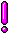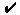WON plate78 | World!OfNumbers[ October 3, 2000 ] Sets of three composites in bi-directional 'Sum Of Prime Factors' progression/retrogression I'd like to submit to the devoted number lovers the following rather hard puzzle( catalogued as Sloane's A057874 ) in the hope of receiving the elusive  4 th set of three composites in bi-directional 'Sum Of Prime Factors' progression/retrogression. A mouthful, I am aware! Hence this jolly table which will clarify the concept better than any words can.Many more triplets have been found [ October, 2000 ]by  Roberto Botrugno  from Italy... read on!
95 174191 298687992 + 5.19 =In the first set you'll notice no doubt six consecutive primes 5, 7, 11, 13, 17 and 19.All the members of the first two sets are semi-primes i.e. having exactly two distinct factors.The third set has a common prime divisor > 2 to all members namely 179.Curious is also the loop taken counterclockwise of the middle digits of the factors of the second set starting from 443. It starts with a 4, then a 5, then a ... up to and then finally a 9 in factor 397. 373 * 467383 * 457397 * 443Common prime divisor of the members of the composite triplet (298687992, 298688708, 298689424) which are in a bi-directional 'sum of prime factors' (i.e., 716) progression/retrogression.Some OEIS entries A050780 - (n + sopf_n = m) and (m - sopf_m = n). Sequence gives values of n. A050781 - (n + sopf_n = m) and (m - sopf_m = n). Sequence gives values of m. A057874 - Sets of three composites in bidirectional 'sum of prime factors' progression/retrogression.A000078Prime Curios!Prime PuzzleWikipedia 78Le Nombre 78Numberland 78```

```

[TOP OF PAGE]

Patrick De Geest - Belgium- Short Bio - Some Pictures